This can be used to simulate fires, seed dispersal, calculation of iterative, concentric landscape values (symmetric or asymmetric) and many other things. Essentially, it starts from a collection of cells (loci) and spreads to neighbours, according to the directions and spreadProb arguments. This can become quite general, if spreadProb is 1 as it will expand from every loci until all cells in the landscape have been covered. With id set to TRUE, the resulting map will be classified by the index of the cell where that event propagated from. This can be used to examine things like fire size distributions. NOTE: See also spread2, which is more robust and can be used to build custom functions. However, under some conditions, this spread function is faster. The two functions can accomplish many of the same things, and key differences are internal.

spread(
landscape,
loci = NA_real_,
persistence = 0,
maxSize = 100000000L,
directions = 8L,
iterations = 1000000L,
returnIndices = FALSE,
returnDistances = FALSE,
mapID = NULL,
id = FALSE,
plot.it = FALSE,
circle = FALSE,
stopRule = NA,
stopRuleBehavior = "includeRing",
allowOverlap = FALSE,
asymmetry = NA_real_,
asymmetryAngle = NA_real_,
quick = FALSE,
neighProbs = NULL,
exactSizes = FALSE,
...
)

# S4 method for RasterLayer
landscape,
loci,
persistence,
maxSize,
directions,
iterations,
lowMemory,
returnIndices,
returnDistances,
mapID,
id,
plot.it,
circle,
stopRule,
stopRuleBehavior,
allowOverlap,
asymmetry,
asymmetryAngle,
quick,
neighProbs,
exactSizes,
.zeroVector,
...
)

## Arguments

landscape A RasterLayer object. This defines the possible locations for spreading events to start and spread into. This can also be used as part of stopRule. A vector of locations in landscape. These should be cell indices. If user has x and y coordinates, these can be converted with cellFromXY. Numeric, or RasterLayer. If numeric of length 1, then this is the global probability of spreading into each cell from a neighbour. If a raster (or a vector of length ncell(landscape), resolution and extent of landscape), then this will be the cell-specific probability. Default is 0.23. If a spreadProbLater is provided, then this is only used for the first iteration. Also called "escape probability". See section on "Breaking out of spread events". A length 1 probability that an active cell will continue to burn, per time step. non-NULL, a RasterLayer object congruent with landscape whose elements are 0,1, where 1 indicates "cannot spread to". Currently not implemented, but identical behaviour can be achieved if spreadProb has zeros in all unspreadable locations. Numeric. Maximum number of cells for a single or all events to be spread. Recycled to match loci length, if it is not as long as loci. See section on Breaking out of spread events. The number of adjacent cells in which to look; default is 8 (Queen case). Can only be 4 or 8. Number of iterations to spread. Leaving this NULL allows the spread to continue until stops spreading itself (i.e., exhausts itself). Logical. If true, then function uses package ff internally. This is slower, but much lower memory footprint. Logical or numeric. If 1 or TRUE, will return a data.table with indices and values of successful spread events. If 2, it will simply return a vector of pixel indices of all cells that were touched. This will be the fastest option. If FALSE, then it will return a raster with values. See Details. Logical. Should the function include a column with the individual cell distances from the locus where that event started. Default is FALSE. See Details. Deprecated. Use id. Logical. If TRUE, returns a raster of events ids. If FALSE, returns a raster of iteration numbers, i.e., the spread history of one or more events. NOTE: this is overridden if returnIndices is TRUE or 1 or 2. If TRUE, then plot the raster at every iteration, so one can watch the spread event grow. Numeric, or RasterLayer. If provided, then this will become the spreadProb after the first iteration. See Details. data.table. This should be the output of a previous call to spread, where returnIndices was TRUE. Default NA, meaning the spread is starting from loci. See Details. Logical. If TRUE, then outward spread will be by equidistant rings, rather than solely by adjacent cells (via directions arg.). Default is FALSE. Using circle = TRUE can be dramatically slower for large problems. Note, this should usually be used with spreadProb = 1. Numeric. A further way to stop the outward spread of events. If circle is TRUE, then it will grow to this maximum radius. See section on Breaking out of spread events. Default is NA. A function which will be used to assess whether each individual cluster should stop growing. This function can be an argument of "landscape", "id", "cells", and any other named vectors, a named list of named vectors, or a named data.frame with column names passed to spread in the .... Default NA, meaning that spreading will not stop as a function of the landscape. See section on "Breaking out of spread events" and examples. Character. Can be one of "includePixel", "excludePixel", "includeRing", or "excludeRing". If stopRule contains a function, this argument is used determine what to do with the cell(s) that caused the rule to be TRUE. See details. Default is "includeRing" which means to accept the entire ring of cells that caused the rule to be TRUE. Logical. If TRUE, then individual events can overlap with one another, i.e., they do not interact (this is slower than if allowOverlap = FALSE). Default is FALSE. A numeric indicating the ratio of the asymmetry to be used. Default is NA, indicating no asymmetry. See details. This is still experimental. Use with caution. A numeric indicating the angle in degrees (0 is "up", as in North on a map), that describes which way the asymmetry is. Logical. If TRUE, then several potentially time consuming checking (such as inRange) will be skipped. This should only be used if there is no concern about checking to ensure that inputs are legal. A numeric vector, whose sum is 1. It indicates the probabilities an individual spread iteration spreading to 1:length(neighProbs) neighbours. Logical. If TRUE, then the maxSize will be treated as exact sizes, i.e., the spread events will continue until they are floor(maxSize). This is overridden by iterations, but if iterations is run, and individual events haven't reached maxSize, then the returned data.table will still have at least one active cell per event that did not achieve maxSize, so that the events can continue if passed into spread with spreadState. Logical. If TRUE, then spreadProb will be rescaled *within* the directions neighbours, such that the sum of the probabilities of all neighbours will be 1. Default FALSE, unless spreadProb values are not contained between 0 and 1, which will force relativeSpreadProb to be TRUE. Additional named vectors or named list of named vectors required for stopRule. These vectors should be as long as required e.g., length loci if there is one value per event. DESCRIPTION NEEDED

## Value

Either a RasterLayer indicating the spread of the process in the landscape or a data.table if returnIndices is TRUE. If a RasterLayer, then it represents every cell in which a successful spread event occurred. For the case of, say, a fire this would represent every cell that burned. If allowOverlap is TRUE, This RasterLayer will represent the sum of the individual event ids (which are numerics seq_along(loci). This will generally be of minimal use because it won't be possible to distinguish if event 2 overlapped with event 5 or if it was just event 7.

If returnIndices is TRUE, then this function returns a data.table with columns:

 id an arbitrary ID 1:length(loci) identifying unique clusters of spread events, i.e., all cells that have been spread into that have a common initial cell. initialLocus the initial cell number of that particular spread event. indices The cell indices of cells that have been touched by the spread algorithm. active a logical indicating whether the cell is active (i.e., could still be a source for spreading) or not (no spreading will occur from these cells).

This will generally be more useful when allowOverlap is TRUE.

## Details

For large rasters, a combination of lowMemory = TRUE and returnIndices = TRUE or returnIndices = 2 will be fastest and use the least amount of memory.

This function can be interrupted before all active cells are exhausted if the iterations value is reached before there are no more active cells to spread into. If this is desired, returnIndices should be TRUE and the output of this call can be passed subsequently as an input to this same function. This is intended to be used for situations where external events happen during a spread event, or where one or more arguments to the spread function change before a spread event is completed. For example, if it is desired that the spreadProb change before a spread event is completed because, for example, a fire is spreading, and a new set of conditions arise due to a change in weather.

asymmetry is currently used to modify the spreadProb in the following way. First for each active cell, spreadProb is converted into a length 2 numeric of Low and High spread probabilities for that cell: spreadProbsLH <- (spreadProb*2) // (asymmetry+1)*c(1,asymmetry), whose ratio is equal to asymmetry. Then, using asymmetryAngle, the angle between the initial starting point of the event and all potential cells is found. These are converted into a proportion of the angle from -asymmetryAngle to asymmetryAngle using: angleQuality <- (cos(angles - rad(asymmetryAngle))+1)/2

These are then converted to multiple spreadProbs by spreadProbs <- lowSpreadProb+(angleQuality * diff(spreadProbsLH)) To maintain an expected spreadProb that is the same as the asymmetric spreadProbs, these are then rescaled so that the mean of the asymmetric spreadProbs is always equal to spreadProb at every iteration: spreadProbs <- spreadProbs - diff(c(spreadProb,mean(spreadProbs)))

## Breaking out of spread events

There are 4 ways for the spread to "stop" spreading. Here, each "event" is defined as all cells that are spawned from a single starting loci. So, one spread call can have multiple spreading "events". The ways outlines below are all acting at all times, i.e., they are not mutually exclusive. Therefore, it is the user's responsibility to make sure the different rules are interacting with each other correctly. Using spreadProb or maxSize are computationally fastest, sometimes dramatically so.

 spreadProb Probabilistically, if spreadProb is low enough, active spreading events will stop. In practice, active spreading events will stop. In practice, this number generally should be below 0.3 to actually see an event stop maxSize This is the number of cells that are "successfully" turned on during a spreading event. This can be vectorized, one value for each event circleMaxRadius If circle is TRUE, then this will be the maximum radius reached, and then the event will stop. This is vectorized, and if length is >1, it will be matched in the order of loci stopRule This is a function that can use "landscape", "id", "cells", or any named vector passed into spread in the .... This can take on relatively complex functions. Passing in, say, a RasterLayer to spread can access the individual values on that arbitrary RasterLayer using "cells". These will be calculated within all the cells of the individual event (equivalent to a "group_by(event)" in dplyr. So, sum(arbitraryRaster[cells]) would sum up all the raster values on the arbitraryRaster raster that are overlaid by the individual event. This can then be used in a logical statement. See examples. To confirm the cause of stopping, the user can assess the values after the function has finished.

The spread function does not return the result of this stopRule. If, say, an event has both circleMaxRadius and stopRule, and it is the circleMaxRadius that caused the event spreading to stop, there will be no indicator returned from this function that indicates which rule caused the stop.

stopRule has many use cases. One common use case is evaluating a neighbourhood around a focal set of points. This provides, therefore, an alternative to the buffer function or focal function. In both of those cases, the window/buffer size must be an input to the function. Here, the resulting size can be emergent based on the incremental growing and calculating of the landscape values underlying the spreading event.

## stopRuleBehavior

This determines how the stopRule should be implemented. Because spreading occurs outwards in concentric circles or shapes, one cell width at a time, there are 4 possible ways to interpret the logical inequality defined in stopRule. In order of number of cells included in resulting events, from most cells to fewest cells:

 "includeRing" Will include the entire ring of cells that, as a group, caused stopRule to be TRUE. "includePixel" Working backwards from the entire ring that caused the stopRule to be TRUE, this will iteratively random cells in the final ring until the stopRule is FALSE. This will add back the last removed cell and include it in the return result for that event. "excludePixel" Like "includePixel", but it will not add back the cell that causes stopRule to be TRUE "excludeRing" Analogous to "excludePixel", but for the entire final ring of cells added. This will exclude the entire ring of cells that caused the stopRule to be TRUE

spread2 for a different implementation of the same algorithm. It is more robust, meaning, there will be fewer unexplainable errors, and the behaviour has been better tested, so it is more likely to be exactly as described under all argument combinations. Also, rings which uses spread but with specific argument values selected for a specific purpose. distanceFromPoints. cir to create "circles"; it is fast for many small problems.

## Examples

library(raster)
library(RColorBrewer)
library(quickPlot)

# Make random forest cover map
set.seed(123)
emptyRas <- raster(extent(0, 1e2, 0, 1e2), res = 1)
hab <- randomPolygons(emptyRas, numTypes = 40)
names(hab) <- "hab"
numCol <- ncol(emptyRas)
numCell <- ncell(emptyRas)
directions <- 8

# Can use transparent as a colour
setColors(hab) <- paste(c("transparent", brewer.pal(8, "Greys")))

# note speedup is equivalent to making pyramids, so, some details are lost
clearPlot()Plot(hab, speedup = 3)# initiate 10 fires
startCells <- as.integer(sample(1:ncell(emptyRas), 100))
fires <- spread(hab, loci = startCells, 0.235, persistence = 0, numNeighs = 2,
mask = NULL, maxSize = 1e8, directions = 8, iterations = 1e6, id = TRUE)

#set colours of raster, including a transparent layer for zeros
setColors(fires, 10) <- c("transparent", brewer.pal(8, "Reds")[5:8])
Plot(fires)Plot(fires, addTo = "hab")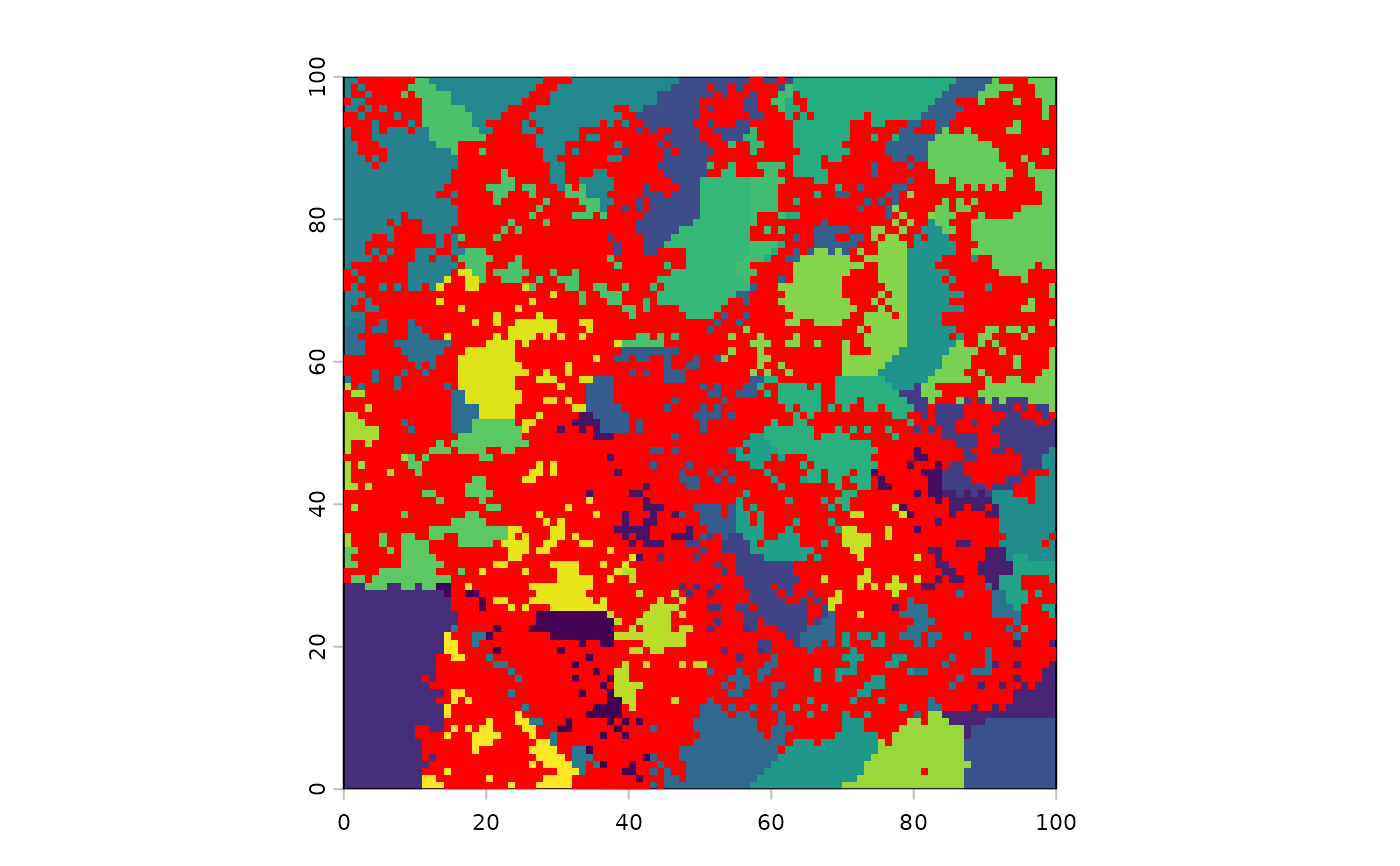#alternatively, set colours using cols= in the Plot function
clearPlot()Plot(hab)Plot(fires) # default colour range makes zero transparent.
# Instead, to give a colour to the zero values, use \code{zero.color=}
cols = colorRampPalette(c("orange", "darkred"))(10), zero.color = "transparent")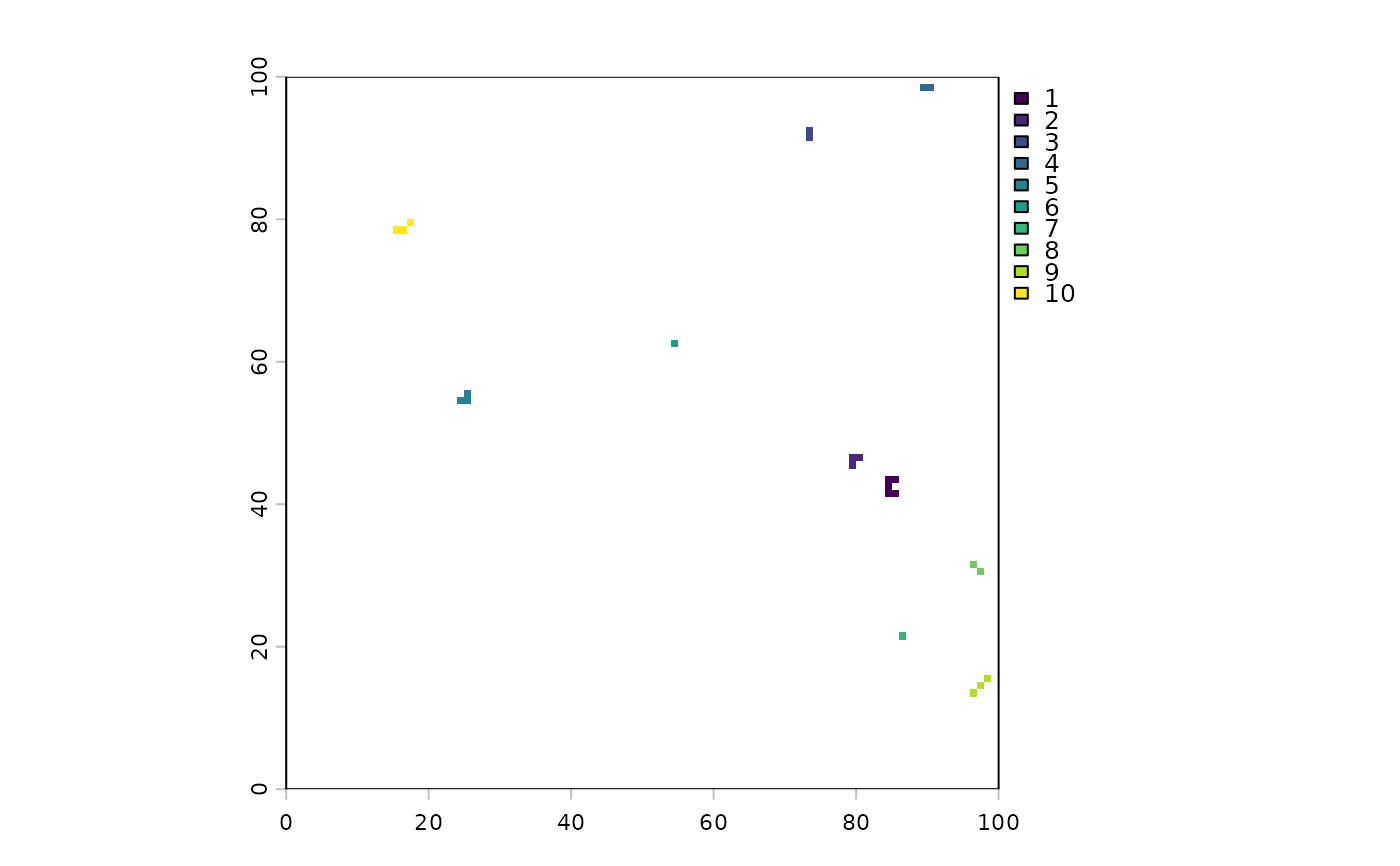hab2 <- hab
Plot(hab2)Plot(fires, addTo = "hab2", zero.color = "transparent",
cols = colorRampPalette(c("orange", "darkred"))(10))# or overplot the original (NOTE: legend stays at original values)
Plot(fires, cols = topo.colors(10), new = TRUE, zero.color = "white")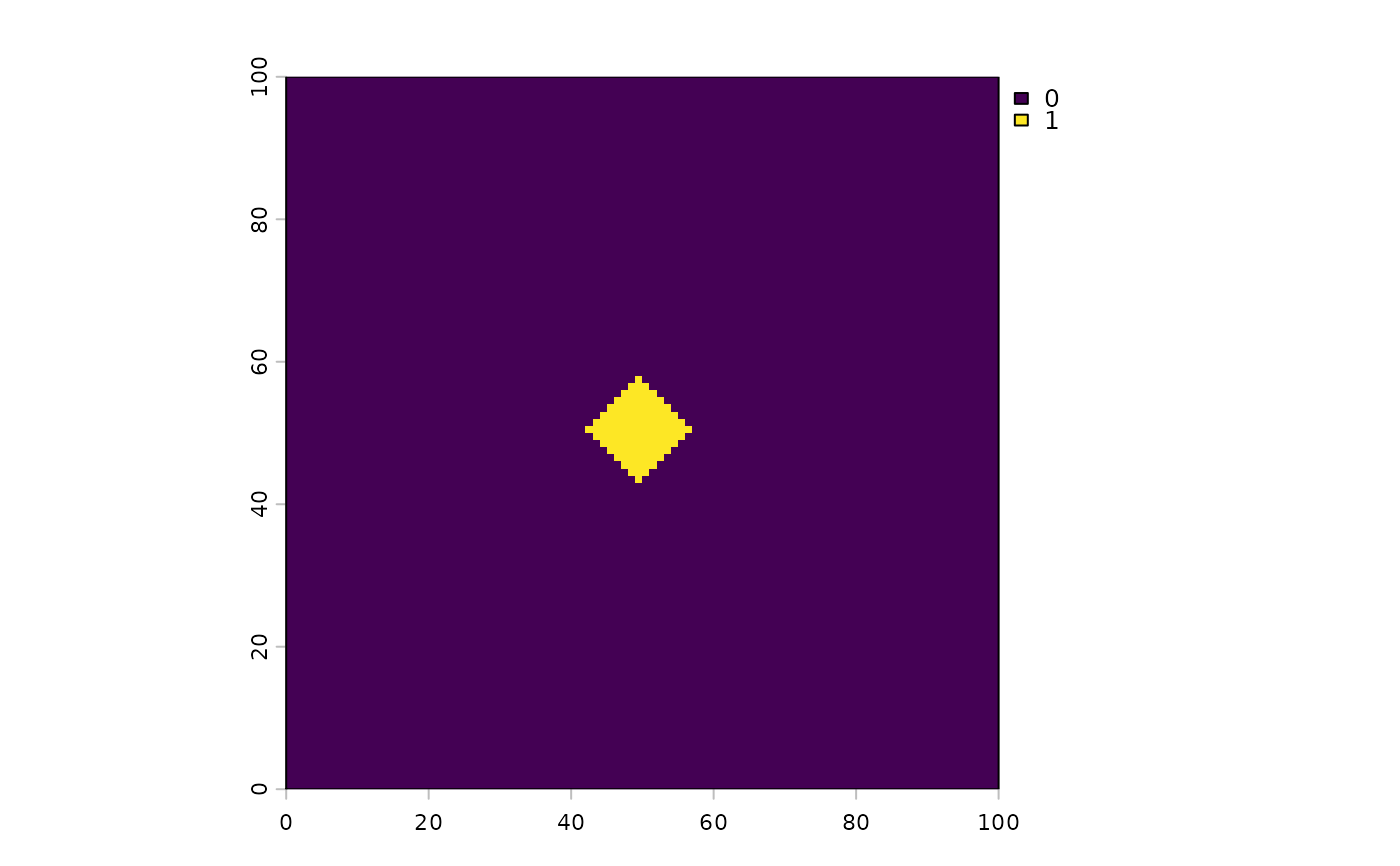##------------------------------------------------------------------------------
## Continue event by passing interrupted object into spreadState
##------------------------------------------------------------------------------

## Interrupt a spread event using iterations - need returnIndices = TRUE to
##  use outputs as new inputs in next iteration
fires <- spread(hab, loci = as.integer(sample(1:ncell(hab), 10)),
returnIndices = TRUE, 0.235, 0, NULL, 1e8, 8, iterations = 3, id = TRUE)
fires[, list(size = length(initialLocus)), by = id]  # See sizes of fires#>     id size
#>  1:  1    1
#>  2:  2   13
#>  3:  3    1
#>  4:  4    1
#>  5:  5    5
#>  6:  6   12
#>  7:  7    1
#>  8:  8   13
#>  9:  9   24
#> 10: 10   18
fires2 <- spread(hab, loci = NA_real_, returnIndices = TRUE, 0.235, 0, NULL,
1e8, 8, iterations = 2, id = TRUE, spreadState = fires)
# NOTE events are assigned arbitrary IDs, starting at 1

fires3 <- spread(hab, loci = as.integer(sample(1:ncell(hab), 10)),
returnIndices = TRUE, 0.235, 0, NULL, 1e8, 8, iterations = 1,
id = TRUE, spreadState = fires)
fires3[, list(size = length(initialLocus)), by = id]  # See sizes of fires#>     id size
#>  1:  1    1
#>  2:  2   12
#>  3:  3    1
#>  4:  4    1
#>  5:  5    5
#>  6:  6   12
#>  7:  7    1
#>  8:  8   14
#>  9:  9   26
#> 10: 10   17# NOTE old ids are maintained, new events get ids begining above previous
# maximum (e.g., new fires 11 to 20 here)

## Use data.table and loci...
fires <- spread(hab, loci = as.integer(sample(1:ncell(hab), 10)),
returnIndices = TRUE, 0.235, 0, NULL, 1e8, 8, iterations = 2, id = TRUE)
fullRas <- raster(hab)
fullRas[] <- 1:ncell(hab)
burned <- fires[active == FALSE]
burnedMap <- rasterizeReduced(burned, fullRas, "id", "indices")

clearPlot()Plot(burnedMap, new = TRUE)####################
## stopRule examples
####################

# examples with stopRule, which means that the eventual size is driven by the values on the raster
#  passed in to the landscape argument
set.seed(1234)
stopRule1 <- function(landscape) sum(landscape) > 50
stopRuleA <- spread(hab, loci = as.integer(sample(1:ncell(hab), 10)), 1, 0, NULL,
maxSize = 1e6, 8, 1e6, id = TRUE, circle = TRUE, stopRule = stopRule1)

set.seed(1234)
stopRule2 <- function(landscape) sum(landscape) > 100
# using stopRuleBehavior = "excludePixel"
stopRuleB <- spread(hab, loci = as.integer(sample(1:ncell(hab), 10)), 1, 0, NULL,
maxSize = 1e6, 8, 1e6, id = TRUE, circle = TRUE, stopRule = stopRule2,
stopRuleBehavior = "excludePixel")

# using stopRuleBehavior = "includeRing", means that end result is slightly larger patches, as a
#  complete "iteration" of the spread algorithm is used.
set.seed(1234)
stopRuleBNotExact <- spread(hab, loci = as.integer(sample(1:ncell(hab), 10)), 1, 0,
NULL, maxSize = 1e6, 8, 1e6, id = TRUE, circle = TRUE,
stopRule = stopRule2)
clearPlot()Plot(stopRuleA, stopRuleB, stopRuleBNotExact)# Test that the stopRules work
# stopRuleA was not exact, so each value will "overshoot" the stopRule, here it was hab>50
foo <- cbind(vals = hab[stopRuleA], id = stopRuleA[stopRuleA > 0]);
tapply(foo[, "vals"], foo[, "id"], sum) # Correct ... all are above 50#>   1   2   3   4   5   6   7   8   9  10
#> 124 154 117  80 185  55  95  95 135 164
# stopRuleB was exact, so each value will be as close as possible while rule still is TRUE
#  Because we have discrete cells, these numbers will always slightly under the rule
foo <- cbind(vals = hab[stopRuleB], id = stopRuleB[stopRuleB > 0]);
tapply(foo[, "vals"], foo[, "id"], sum) # Correct ... all are above 50#>  1  2  3  4  5  6  7  8  9 10
#> 88 74 99 96 74 99 95 95 81 90
# stopRuleB_notExact will overshoot
foo <- cbind(vals = hab[stopRuleBNotExact], id = stopRuleBNotExact[stopRuleBNotExact > 0]);
tapply(foo[, "vals"], foo[, "id"], sum) # Correct ... all are above 50#>   1   2   3   4   5   6   7   8   9  10
#> 124 154 117 208 185 137 247 247 135 164
# Cellular automata shapes
# Diamonds - can make them with: a boolean raster, directions = 4,
#    stopRule in place, spreadProb = 1
diamonds <- spread(hab > 0, spreadProb = 1, directions = 4, id = TRUE, stopRule = stopRule2)

clearPlot()Plot(diamonds)# Squares - can make them with: a boolean raster, directions = 8,
#    stopRule in place, spreadProb = 1
squares <- spread(hab > 0, spreadProb = 1, directions = 8, id = TRUE, stopRule = stopRule2)
Plot(squares)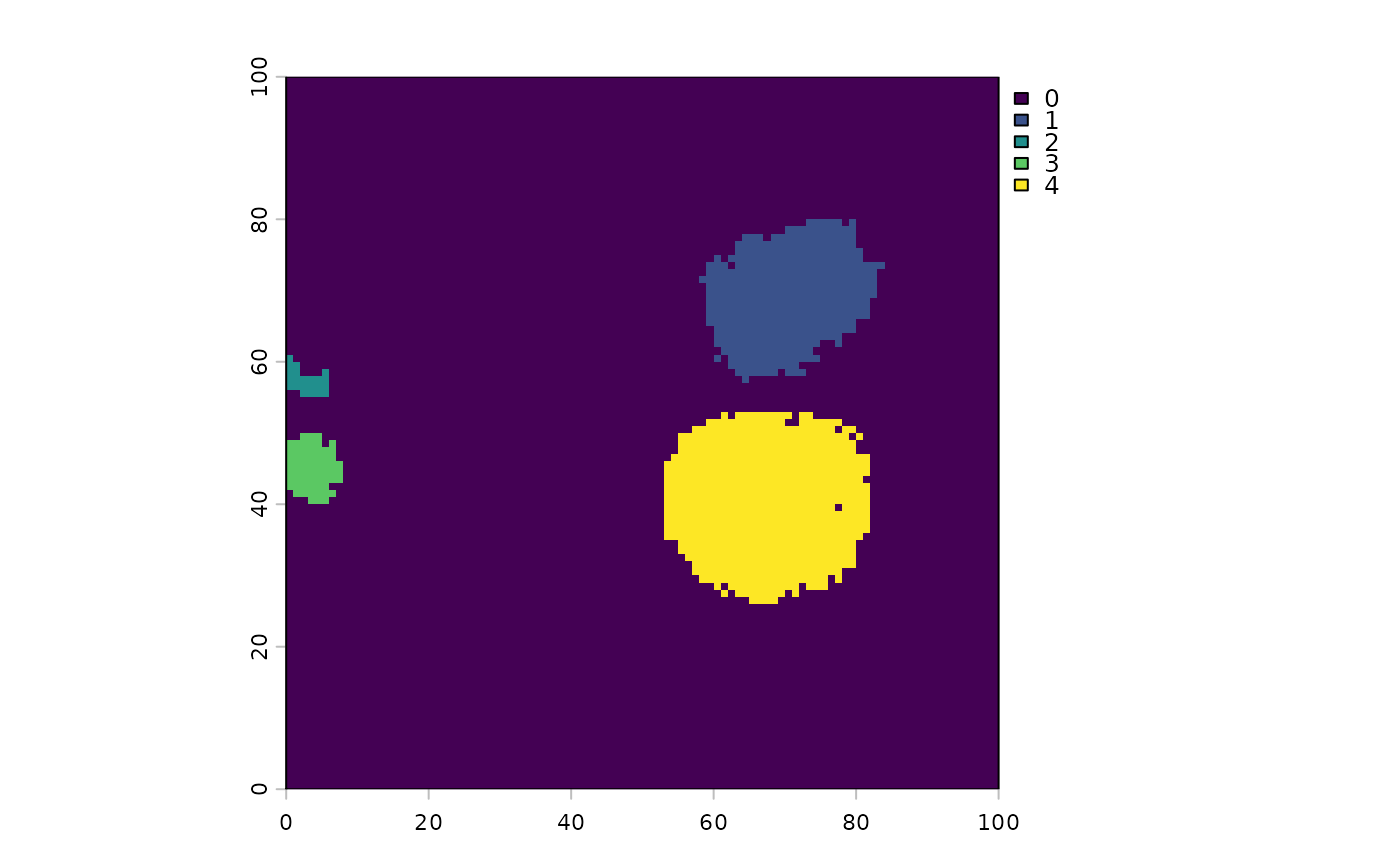# Interference shapes - can make them with: a boolean raster, directions = 8,
#    stopRule in place, spreadProb = 1
stopRule2 <- function(landscape) sum(landscape) > 200
loci = (ncell(hab) - ncol(hab)) / 2 + c(4, -4),
directions = 4, id = TRUE, stopRule = stopRule2)
clearPlot()Plot(squashedDiamonds)# Circles with spreadProb < 1 will give "more" circular shapes, but definitely not circles
stopRule2 <- function(landscape) sum(landscape) > 200
seed <- sample(1e4, 1)
set.seed(seed)
loci = (ncell(hab) - ncol(hab)) / 2 + c(4, -4),
directions = 8, id = TRUE, circle = TRUE)#, stopRule = stopRule2)
set.seed(seed)
loci = (ncell(hab) - ncol(hab)) / 2 + c(4, -4),
directions = 8, id = TRUE)#, stopRule = stopRule2)

clearPlot()Plot(circlish, regularCA)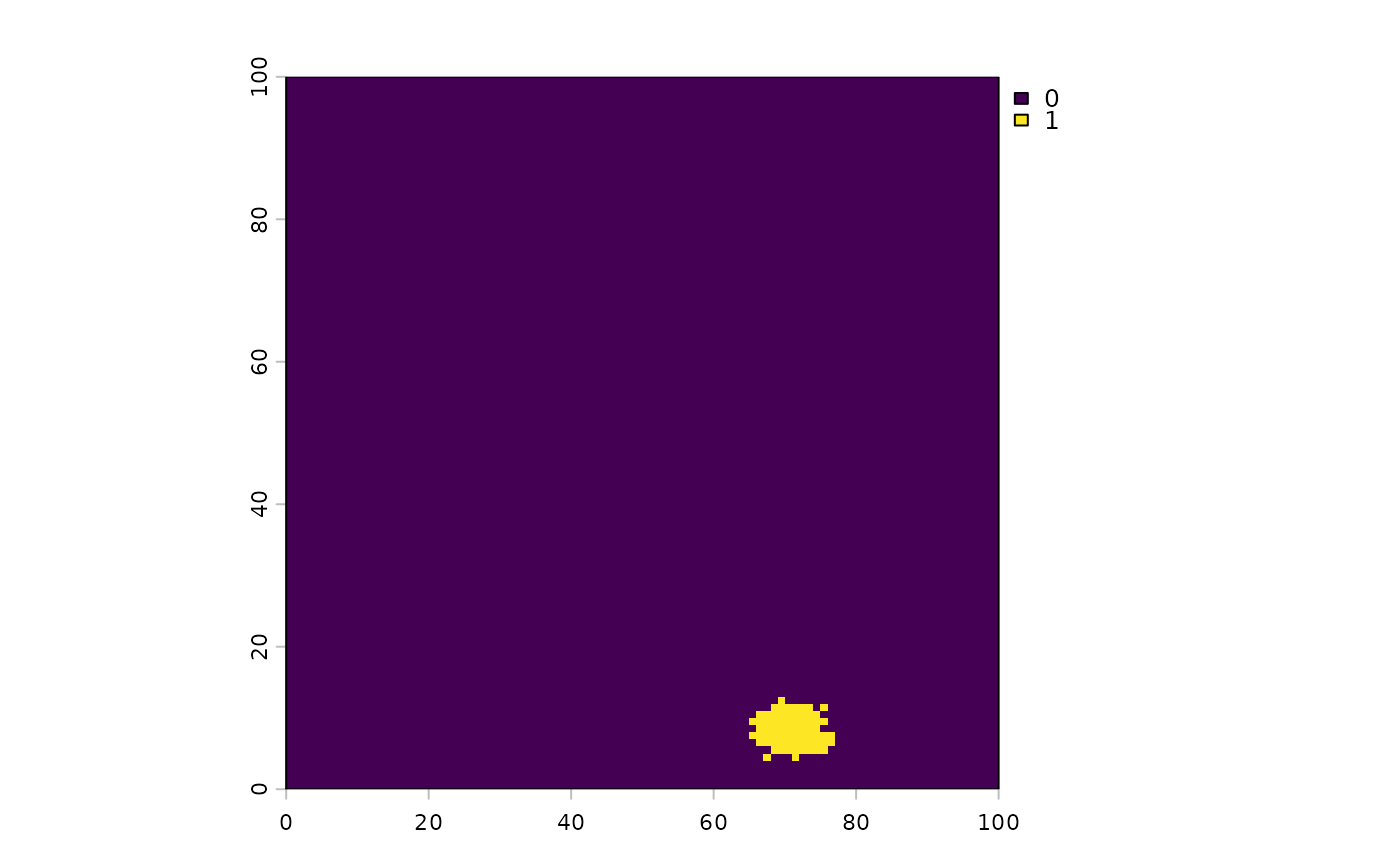####################
# complex stopRule
####################

initialLoci <- sample(seq_len(ncell(hab)), 2)
endSizes <- seq_along(initialLoci) * 200

# Can be a function of landscape, id, and/or any other named
stopRule3 <- function(landscape, id, endSizes) sum(landscape) > endSizes[id]

twoCirclesDiffSize <- spread(hab, spreadProb = 1, loci = initialLoci, circle = TRUE,
directions = 8, id = TRUE, stopRule = stopRule3,
endSizes = endSizes, stopRuleBehavior = "excludePixel")

# or using named list of named elements:
twoCirclesDiffSize2 <- spread(hab, spreadProb = 1, loci = initialLoci, circle = TRUE,
directions = 8, id = TRUE, stopRule = stopRule3,
vars = list(endSizes = endSizes), stopRuleBehavior = "excludePixel")

identical(twoCirclesDiffSize, twoCirclesDiffSize2) ## TRUE#>  TRUE
clearPlot()Plot(twoCirclesDiffSize)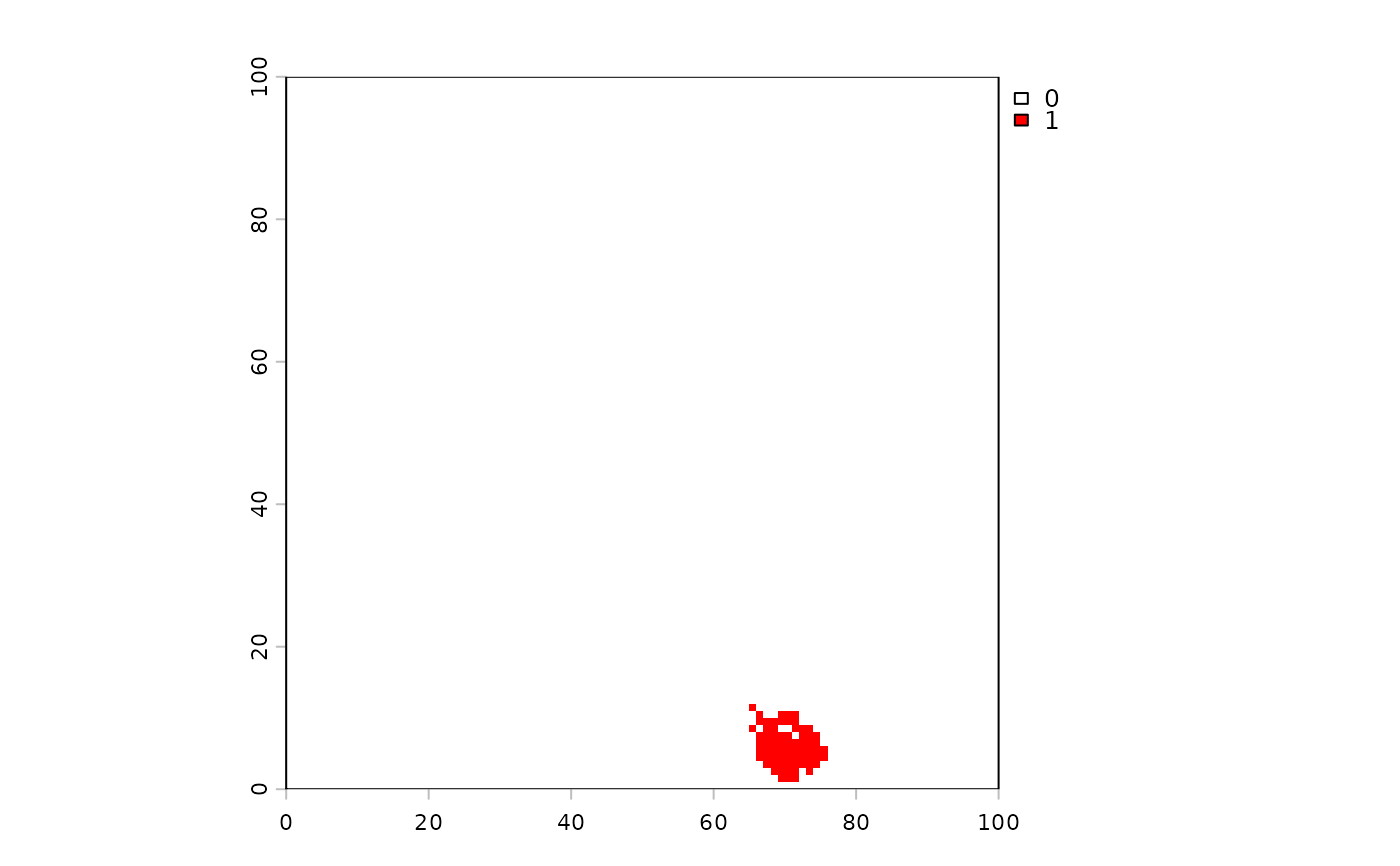cirs <- getValues(twoCirclesDiffSize)
vals <- tapply(hab[twoCirclesDiffSize], cirs[cirs > 0], sum)

# Stop if sum of landscape is big or mean of quality is too small
quality <- raster(hab)
quality[] <- runif(ncell(quality), 0, 1)
stopRule4 <- function(landscape, quality, cells) {
(sum(landscape) > 20) | (mean(quality[cells]) < 0.3)
}

twoCirclesDiffSize <- spread(hab, spreadProb = 1, loci = initialLoci, circle = TRUE,
directions = 8, id = TRUE, stopRule = stopRule4,
quality = quality, stopRuleBehavior = "excludePixel")

##############
# allowOverlap
##############
## TODO: fix error when allowOverlap = TRUE
# set.seed(3113)
# initialLoci <- as.integer(sample(1:ncell(hab), 10))
#
# # using "landscape", "id", and a variable passed in
# maxVal <- rep(500, length(initialLoci))
#
# # define stopRule
# stopRule2 <- function(landscape, id, maxVal) sum(landscape) > maxVal[id]
# circs <- spread(hab, spreadProb = 1, circle = TRUE, loci = initialLoci, stopRule = stopRule2,
#                 id = TRUE, allowOverlap = TRUE, stopRuleBehavior = "includeRing",
#                 maxVal = maxVal, returnIndices = TRUE)
# (vals <- tapply(hab[circs$indices], circs$id, sum))
# vals <= maxVal ## all TRUE
# overlapEvents <- raster(hab)
# overlapEvents[] <- 0
# toMap <- circs[, sum(id), by = indices]
# overlapEvents[toMap$indices] <- toMap$V1
#
# clearPlot()
# Plot(overlapEvents)

## Using alternative algorithm, not probabilistic diffusion
## Will give exactly correct sizes, yet still with variability
## within the spreading (i.e., cells with and without successes)
seed <- sample(1e6, 1)
set.seed(seed)
startCells <- startCells[1:4]
maxSizes <- rexp(length(startCells), rate = 1 / 500)
fires <- spread(hab, loci = startCells, 1, persistence = 0,
neighProbs = c(0.5, 0.5, 0.5) / 1.5,
mask = NULL, maxSize = maxSizes, directions = 8,
iterations = 1e6, id = TRUE, plot.it = FALSE, exactSizes = TRUE);
all(table(fires[fires > 0][]) == floor(maxSizes))#>  TRUE
dev()
clearPlot()Plot(fires, new = TRUE, cols = c("red", "yellow"), zero.color = "white")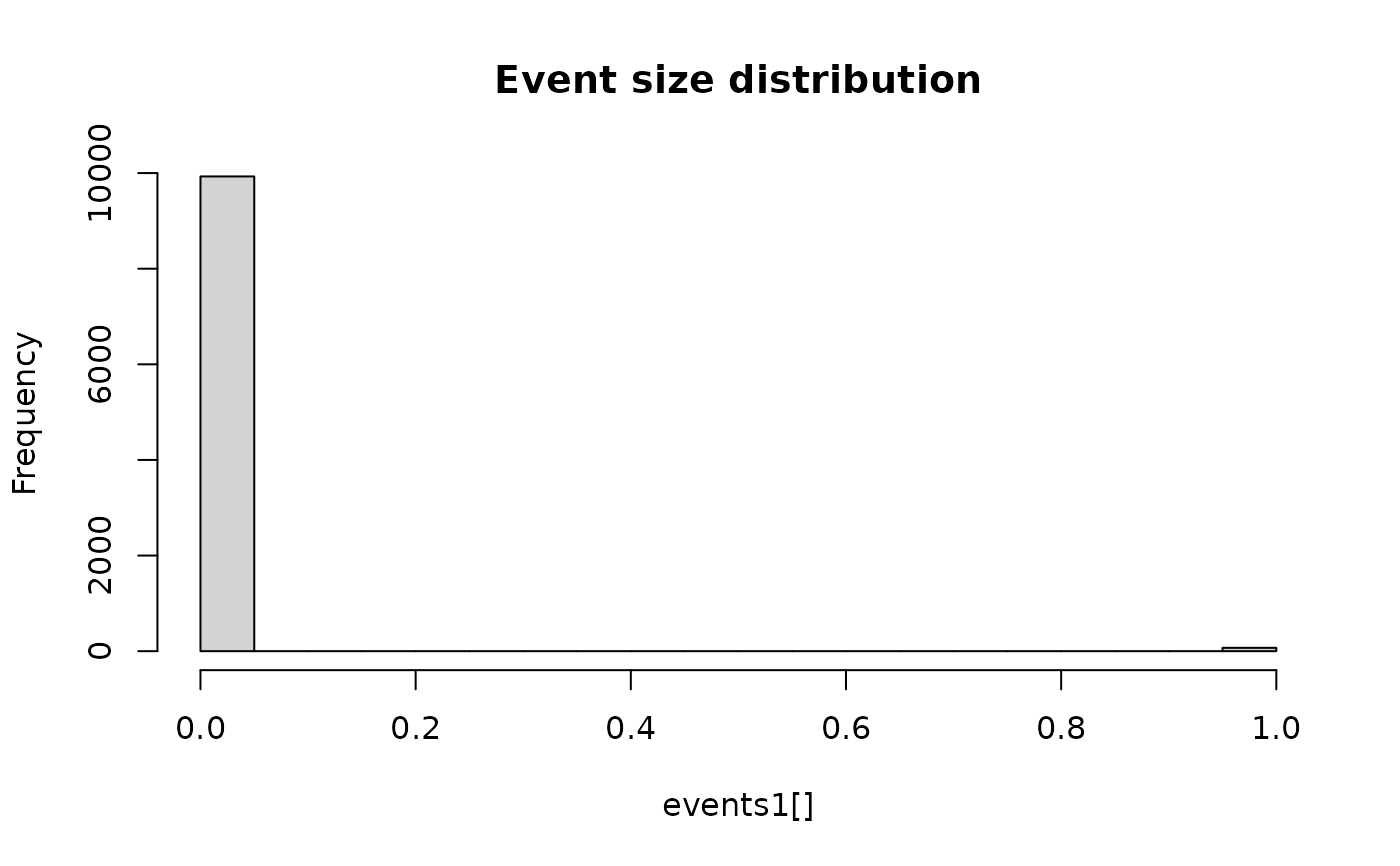Plot(hist(table(fires[][fires[] > 0])), title = "fire size distribution")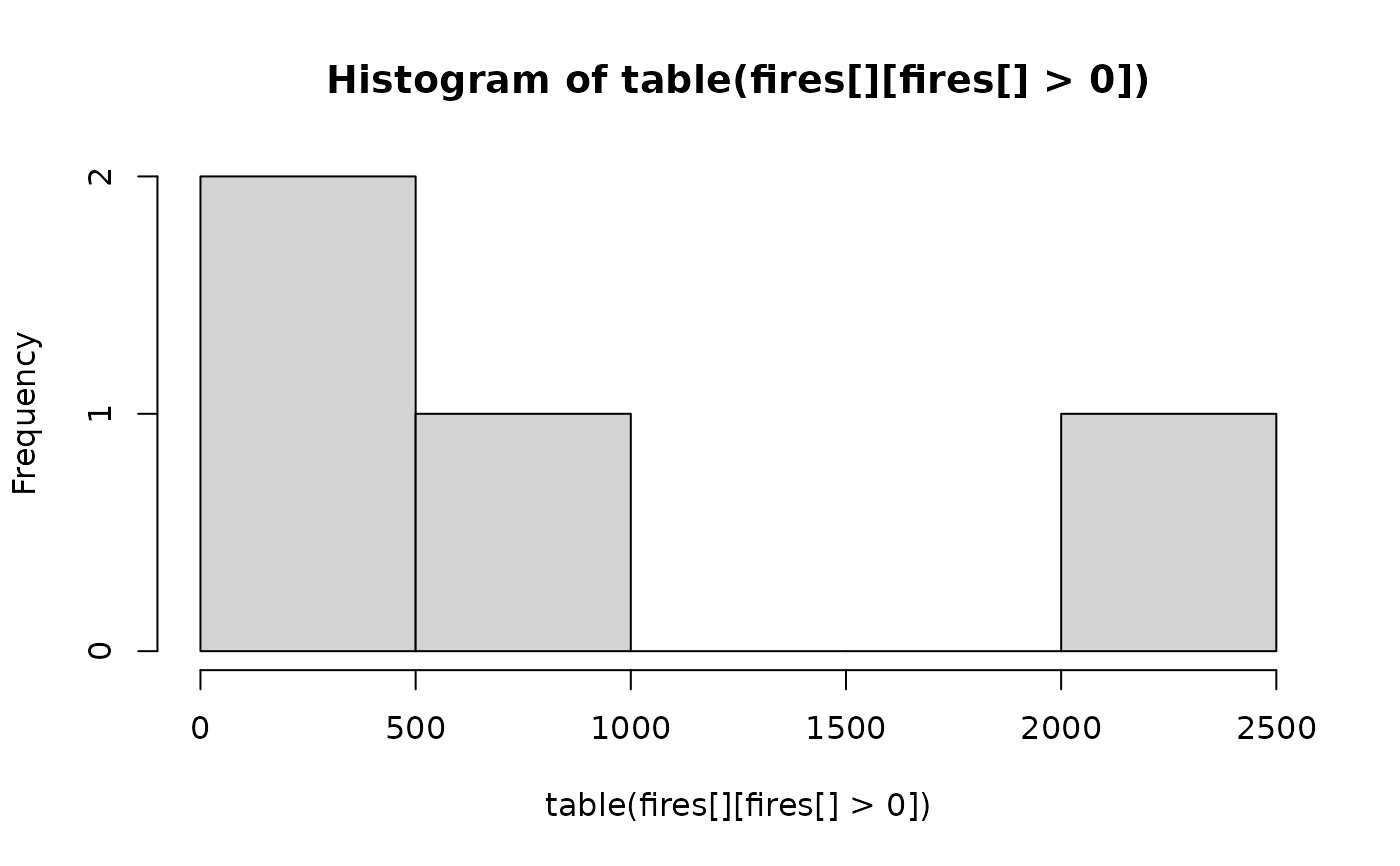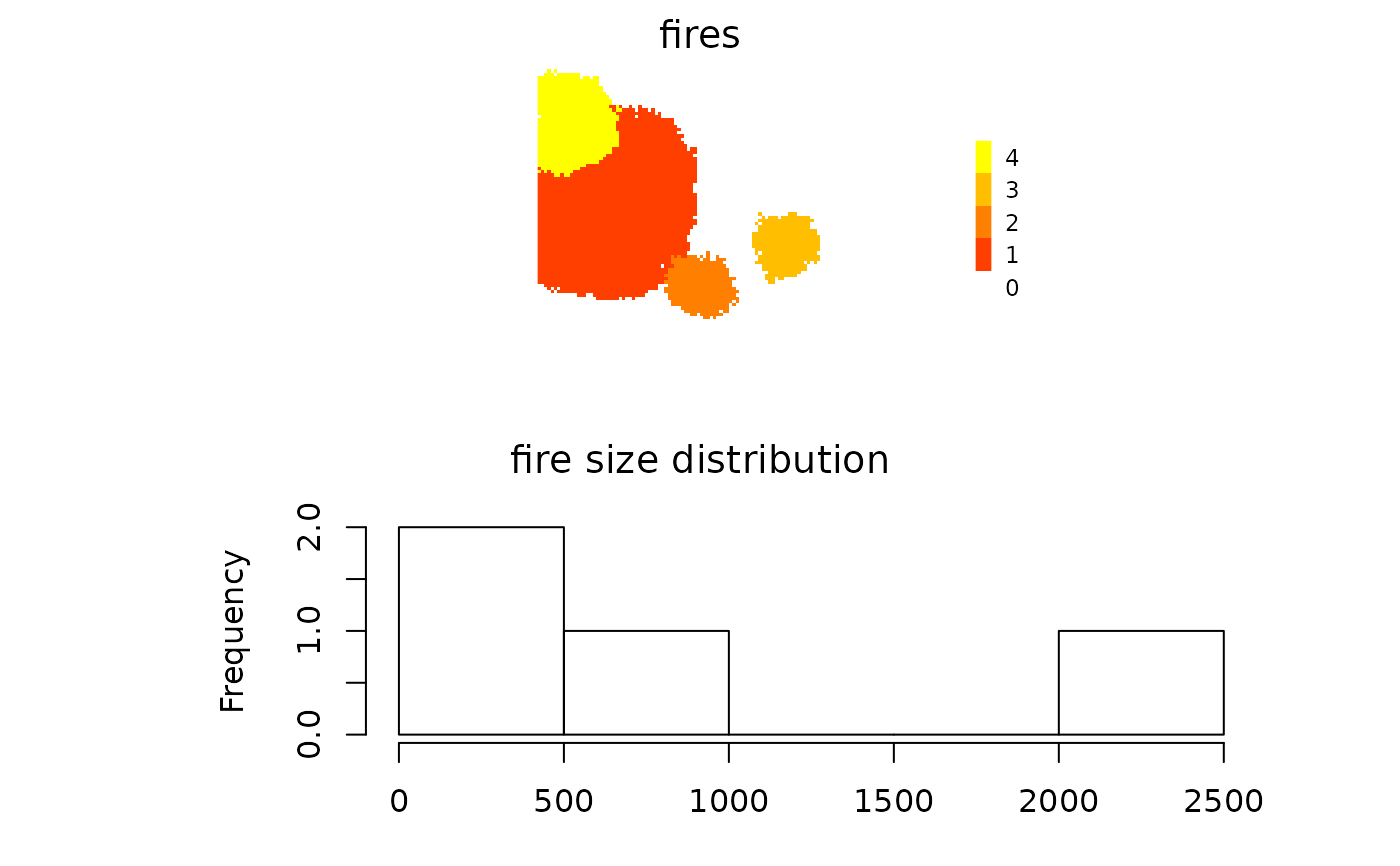##  (shown here because because spreadProb raster is not a probability).
##  Here, we force the events to grow, choosing always 2 neighbours,
##  according to the relative probabilities contained on hab layer.
##
## Note: neighProbs = c(0,1) forces each active pixel to move to 2 new pixels
## (prob = 0 for 1 neighbour, prob = 1 for 2 neighbours)
##
## Note: set hab3 to be very distinct probability differences, to detect spread
##  differences
hab3 <- (hab < 20) * 200 + 1
seed <- 643503
set.seed(seed)
sam <- sample(which(hab3[] == 1), 1)
set.seed(seed)
events1 <- spread(hab3, spreadProb = hab3, loci = sam, directions = 8,
neighProbs = c(0, 1), maxSize = c(70), exactSizes = TRUE)

# Compare to absolute probability version
set.seed(seed)
events2 <- spread(hab3, id = TRUE, loci = sam, directions = 8,
neighProbs = c(0, 1), maxSize = c(70), exactSizes = TRUE)

clearPlot()Plot(events1, new = TRUE, cols = c("red", "yellow"), zero.color = "white")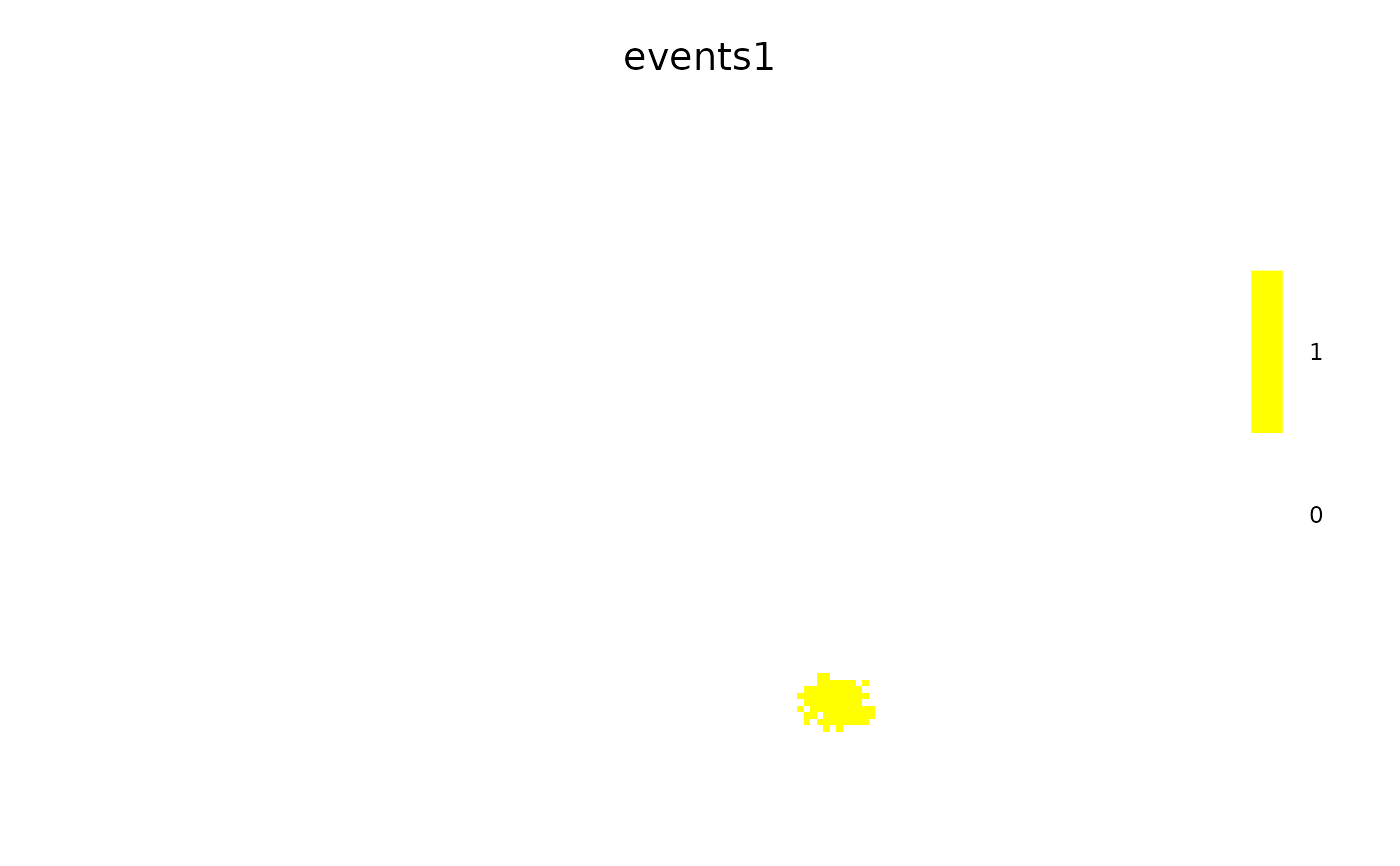Plot(events2, new = TRUE, cols = c("red", "yellow"), zero.color = "white")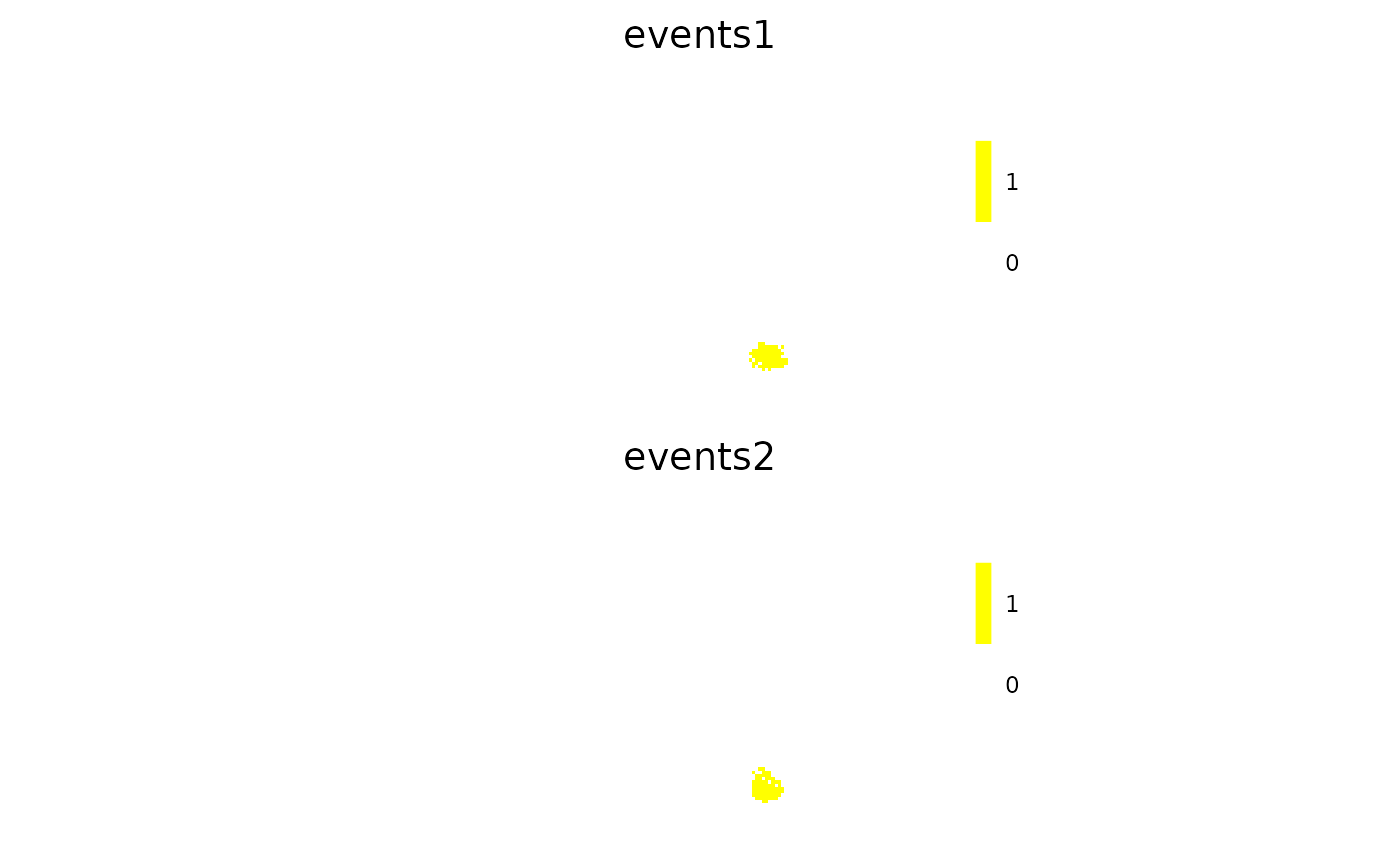Plot(hist(table(events1[][events1[] > 0]), breaks = 30), title = "Event size distribution")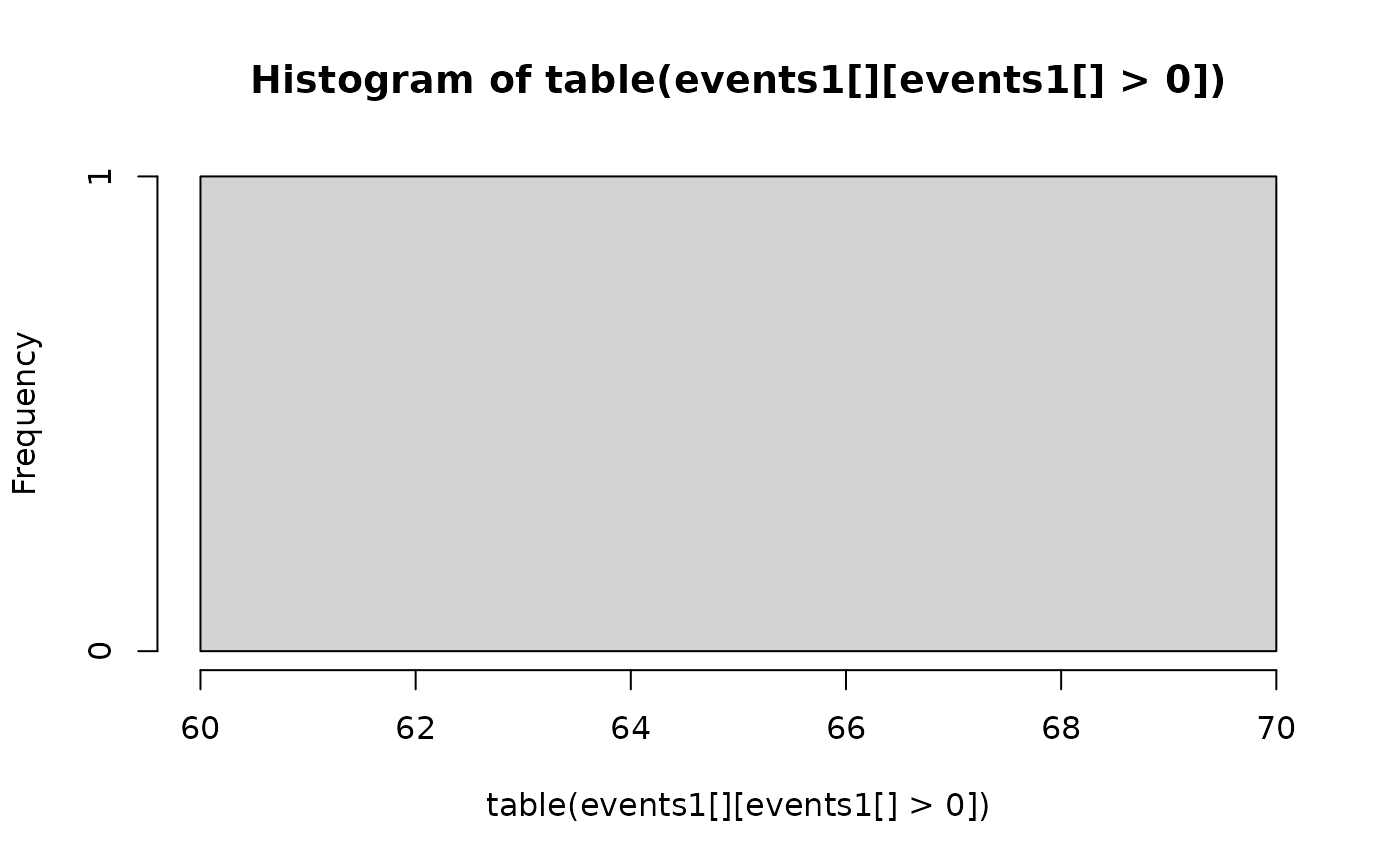# Check that events1 resulted in higher hab3 pixels overall

# Compare outputs -- should be more high value hab pixels spread to in event1
#  (randomness may prevent this in all cases)
hab3[events1[] > 0]#>   201 201 201 201   1   1 201 201 201   1   1 201 201   1   1   1   1 201 201
#>  201 201   1   1   1   1 201 201 201 201 201   1   1   1   1 201 201 201 201
#>    1   1   1   1   1 201 201 201 201   1   1   1   1   1 201 201 201   1   1
#>    1   1 201 201 201 201   1   1   1 201   1   1   1hab3[events2[] > 0]#>     1   1   1 201 201   1   1   1   1   1 201 201   1   1   1   1   1 201 201
#>    1   1   1   1   1   1 201 201   1   1   1   1   1 201 201   1   1   1   1
#>    1 201   1   1   1   1   1   1   1   1 201   1   1   1   1   1   1   1 201
#>    1   1   1   1   1   1   1   1   1   1   1   1   1
sum(hab3[events1[] > 0]) >= sum(hab3[events2[] > 0]) ## should be usually TRUE#>  TRUE